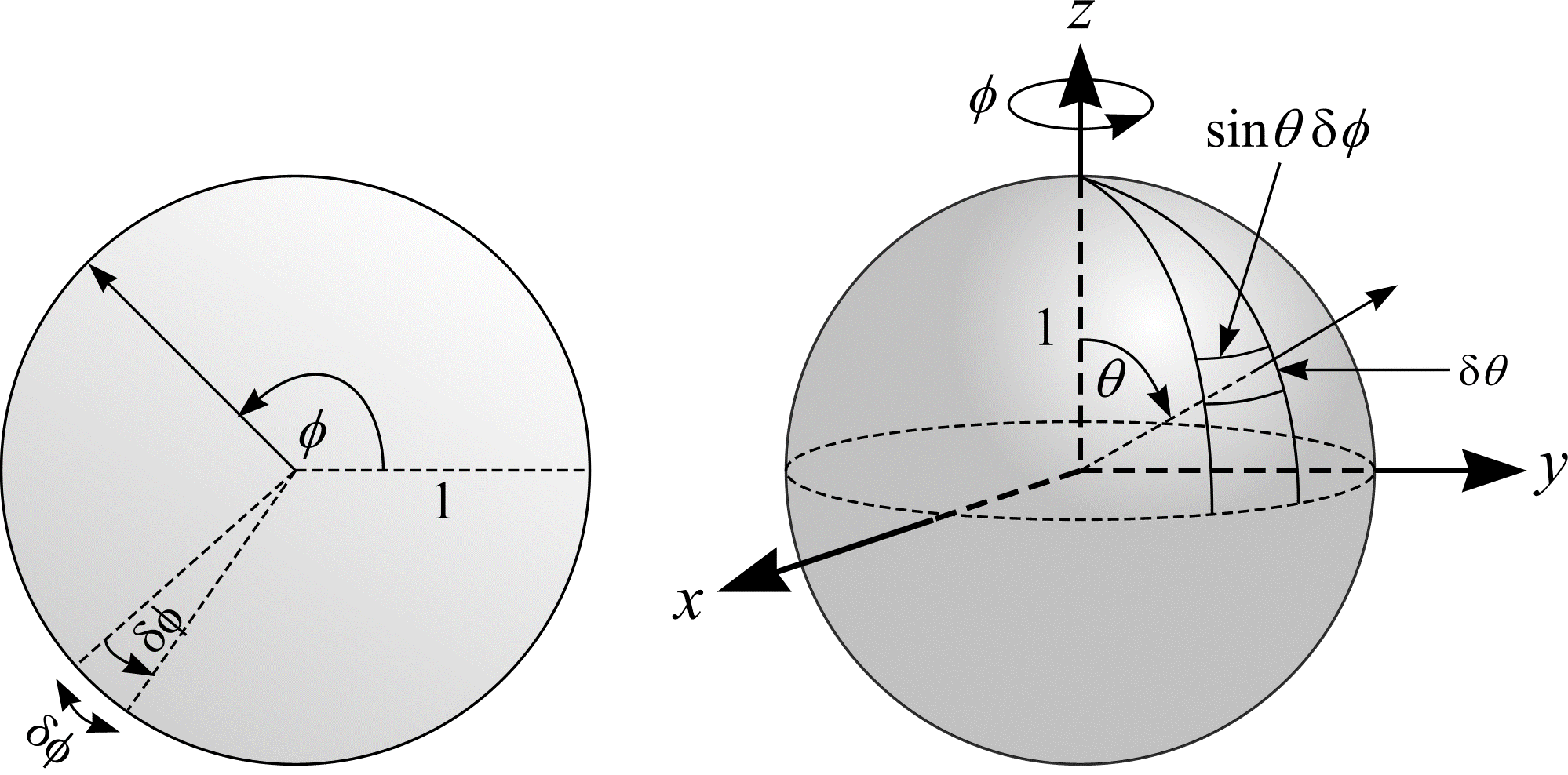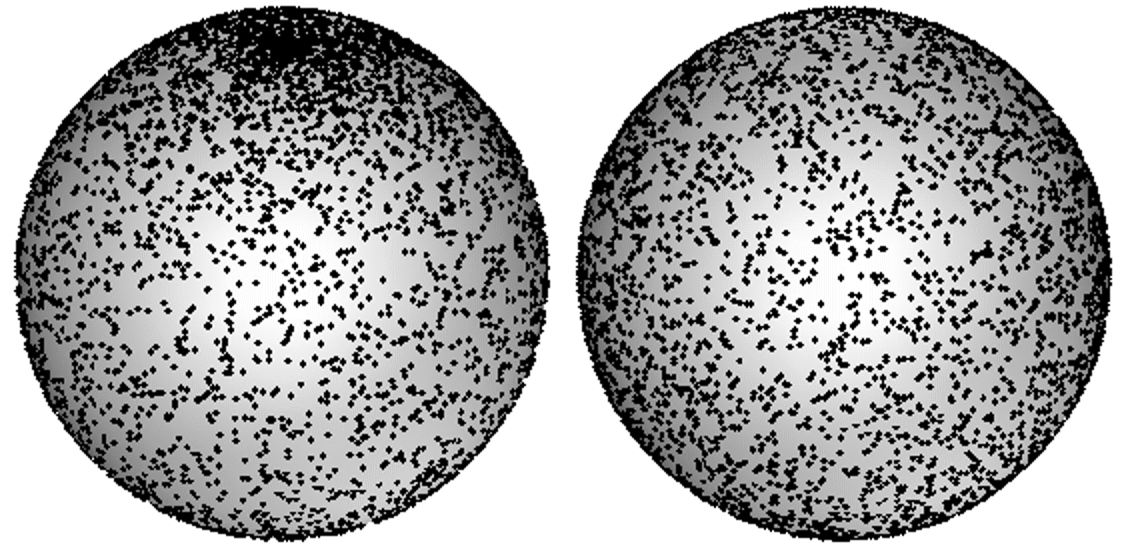# Random position and orientation

An orientation in the plane can be represented by points on the boundary of a circle. An orientation is simply an angle $$\Phi \in [0, 2\pi)$$. The orientation is said to be isotropic random (IR) if and only if the angle $$\Phi$$ is equally likely, i.e. uniform random (UR), in the interval $$[0, 2\pi)$$ (written $$\Phi \in \mathrm{UR}[0, 2\pi))$$. An equivalent statement is that the orientation, $$\Phi$$, is IR if and only if the density function of $$\Phi$$ is constant.

To determine the density function of $$\Phi$$, consider an arc on the unit circle given by $$\{\Phi : \phi \le \Phi \le \phi + \delta\phi\}$$. The arc, of length $$\delta\phi$$, is shown in Figure 6(a). The probability of an orientation falling inside the arc is

\begin{align} \mathbf{P}(\phi \le \Phi \le \phi + \delta\phi) &= \frac{\text{boundary length of arc}}{\text{boundary length of circle}} \\ &= \frac{\delta\phi}{2\pi} \end{align} \tag{23}
$$\mathbf{P}(\phi \le \Phi \le \phi + \delta\phi) = \frac{\text{boundary length of arc}}{\text{boundary length of circle}} = \frac{\delta\phi}{2\pi}$$
$$(23)$$

Equations (7) and (23) imply the density function is $$1/2\pi$$. Furthermore, the function is constant.

The idea can be extended to three dimensions. Orientations in $$\real^{3}$$ can be represented by points on the surface of the unit sphere. Figure 6(b) shows how points on the unit sphere can be obtained by choosing $$\Phi \in [0, 2\pi)$$ and $$\Theta \in [0, \pi)$$. To determine an IR orientation, choose a point uniform randomly on the surface of the sphere (so that regions of equal area will have the same probability to contain the point). It does not suffice to choose $$\Phi$$ and $$\Theta$$ uniform randomly in the intervals $$[0, 2\pi)$$ and $$[0, \pi)$$ respectively. Figure 7(a) shows 10,000 orientations chosen this way. The orientations cluster about the north and south poles.(a)
(b)

Figure 6: (a) An orientation in the plane (represented by the angle $$\phi$$). (b) An orientation in $$\real^{3}$$ (represented by the angles $$\phi$$ and $$\theta$$).

Consider a surface patch on the unit sphere given by $$\{(\Phi, \Theta) : \phi \le \Phi \le \phi + \delta\phi, \theta \le \Theta \le \theta + \delta\theta\}$$. For $$\delta\phi$$ and $$\delta\theta$$ small, the patch approximates a rectangle with dimensions $$\sin(\theta)\delta\phi$$ by $$\delta\theta$$. Suppose $$\Phi$$ and $$\Theta$$ are chosen uniform randomly in the intervals $$[0, 2\pi)$$ and $$[0, \pi)$$ respectively. The probability of the chosen orientation falling inside the patch is

\begin{align} \mathbf{P}\left( \begin{align*} & \phi \le \Phi \le \phi + \delta\phi, \\ & \theta \le \Theta \le \theta + \delta\theta \end{align*} \right) &= \frac{\text{Surface area of patch}}{\text{Surface area of sphere}} \\ &= \frac{\sin(\theta) \delta\phi \delta\theta}{4\pi} \end{align} \tag{24}
$$\mathbf{P}( \phi \le \Phi \le \phi + \delta\phi, \theta \le \Theta \le \theta + \delta\theta ) = \frac{\text{Surface area of patch}}{\text{Surface area of sphere}} = \frac{\sin(\theta) \delta\phi \delta\theta}{4\pi}$$
$$(24)$$

Equations (11) and (24) imply the joint density function of $$\Phi$$ and $$\Theta$$ is $$\sin(\theta)/4\pi$$. To pick IR orientations the density function must remain constant. Clearly $$\mathbf{P}(\delta\theta) = \sin(\theta)\delta\theta$$. Consider the sine-weighting or change of variable $$\theta = \arccos(1 - 2u)$$, where $$u$$ is uniform random in the interval $$[0, 1)$$. Differentiating,

$$\mathrm{d}\theta = -\frac{1}{\sqrt{1 - u^{2}}}\mathrm{d}u$$

and $$\sin(\theta)$$ becomes $$\sin(\arccos(1 - 2u)) = \sqrt{1 - u^{2}}$$. Now $$\mathbf{P}(\delta\theta) = \sin(\theta)\delta\theta = -\delta u$$. Figure 7(b) shows 10,000 IR orientations.

An object (such as a straight line or curve) in some domain $$D \subset \real^{2}$$ is said to be Isotropic Uniform Random (IUR), if it is IR and has UR position within $$D$$. The latter condition holds if a single identifiable point $$P$$ on the object has UR position within $$D$$. This is equivalent to asking that the coordinates of $$P$$ be chosen so that regions of equal area have the same probability of containing $$P$$. Similarly an object such as a plane in some domain $$D \subset \real^{3}$$ is said to be IUR if it is IR and has UR position within $$D$$.(a)
(b)

Figure 7: 10,000 orientations chosen (a) non-isotropically and (b) isotropically.